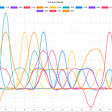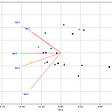# Math for Data Science — Lecture 02 Elementary Row Operations, RREF + Rank via Python

In our previous Lecture 01, we studied about Matrix Operations (Initialization, Vector, Multiplication, Transpose, Special Matrix) https://medium.com/math-for-data-science/math-for-data-science-bits-wilp-program-58417c654665

In this lecture, we will study Elementary row operations to find solution of linear equations. To find same, need to convert matrix into REF (Row Echelon Form) and then to RREF (Reduced row echelon form) via Python.

REF (Row Echelon Form)

• Any rows of all zeros are below any other non zero rows
• Each Leading entry of a row is in a column to the right of the leading entry of the row above it
• All entries in a column below a leading entry are zeros

RREF (Row Reduced Echelon Form)

• Matrix should be in Echelon Form
• Leading entry in each row is 1
• Each leading 1 is the only non zero entry in its column
`## CODE TO GET RREF for Given Matrix -- Reference (https://titanwolf.org/Network/Articles/Article?AID=67790a85-0a2a-4ea2-a6d8-f94325641bc8)def ToReducedRowEchelonForm(M):    ## If M is empty, no need to proceed, and just return    if not M: return    ## if rows are less than column, lead variable used to check that, for every row increment, lead incremented by 1 and if its value greater than or equal to column count, return        lead = 0    ## No of rows in Matrix    rowCount = len(M)    ## No of columns in Matrix    columnCount = len(M)    ## Iterating row of Matrix    for r in range(rowCount):        if lead >= columnCount:            return        i = r        ## If leading element of that row itself 0, check next row's leading element if its zero or not        while M[i][lead] == 0:            i += 1            if i == rowCount:                i = r                lead += 1                if columnCount == lead:                    return        ## Swap Rows i and r --> will happen only if lead element M[i][lead] equal to 0 and i is not equal to rowCount        M[i],M[r] = M[r],M[i]        lv = M[r][lead]        ## Making Lead Entry -- 1        M[r] = [ mrx / float(lv) for mrx in M[r]]        ## Each column will have single Non-Zero entry        for i in range(rowCount):            if i != r:                lv = M[i][lead]                M[i] = [ iv - lv*rv for rv,iv in zip(M[r],M[i])]        lead += 1    return M`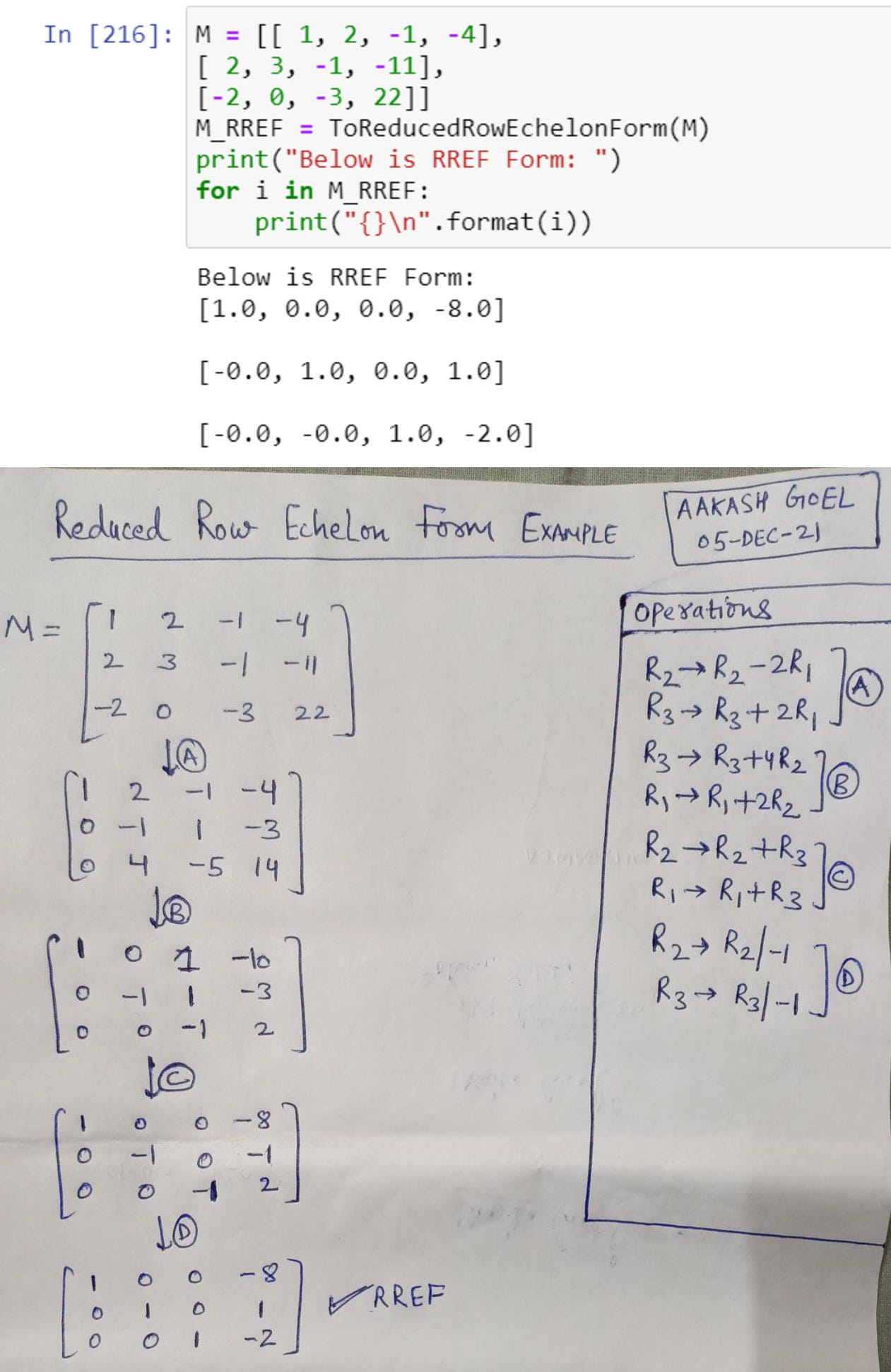Image 03: Output of code — RREF (Via Python and hand)

However, RREF of matrix can also be computed using library name sympy.

Rank of Matrix

• Number of non-zero row in the RREF of matrix

As you can in above Image 04: RREF via Sympy Library, last row of RREF is zero and number of non-zero rows is 2. Hence, Rank of Matrix is 2.

`## CODE TO FIND RANK OF MATRIX# import sympy from sympy import *   M = Matrix([[1, 0, 1, 3], [2, 3, 4, 7], [-1, -3, -3, -4]])print("Matrix : {} ".format(M))   # Use sympy.rref() method M_RREF = M.rref()print(M_RREF)print("Rank of Matrix {}".format(len(np.nonzero(M_RREF))))### OUTPUT OF ABOVE CODEMatrix : Matrix([[1, 0, 1, 3], [2, 3, 4, 7], [-1, -3, -3, -4]]) Matrix([[1, 0, 1, 3], [0, 1, 2/3, 1/3], [0, 0, 0, 0]])Rank of Matrix 2`

Matrix Form of Linear System

• A linear system is consistent if rank(A) = rank(A|b) and inconsistent if rank(A) != rank(A|b)
• If rank(A) = rank(A|b) < no of unknowns → Overdetermined (Infinite Solutions)
• A consistent system has at least one solution (thus, one solution or infinitely many solutions), but inconsistent has no solutions at all, as x1 + x2 = 1, x1 + x2 = 0.

Gauss Elimination

• Forward Elimination → Convert Matrix into Upper Triangular Matrix. Swap rows to avoid round off error i.e. find row with max value of pivot. Pivot is element that is first entry in row of upper triangular matrix.
• Back Substitution → Use to find value of variables

Operation Count — Gauss Elimination

• Elimination
• Back Substitution

As we have already seen that Elimination takes too much time, LU Factorization came for rescue.

LU Factorization

Write Square matrix A as (A = LU)

• Doolittle’s Method
• Crout’s Method
• Cholesky’s Method:

Hurray !! You completed 2nd Lecture.

--

--

--

## More from Math for data science

Idea is to understand math for data science from intuition and code (mainly python)

## Best Themes Visual Studio Code For Developers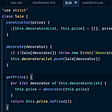## Experimental: Syntax AI with React.js## Marvin — A Machine Learning based approach to Performance Artist Recommendation — Part II## Comparison Inheritance and Polymorphism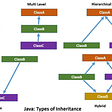## TryHackMe : RootMe Writeup## A Comprehensive Introduction to Binary Search Algorithm## Merging too many small files into fewer large files using Apache Spark in Datalake## How to use Serverless locally with Webpack and Docker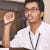## Aakash Goel

Senior Data Scientist @ Fractal Analytics

## Anomaly Detection using AWK & Bash